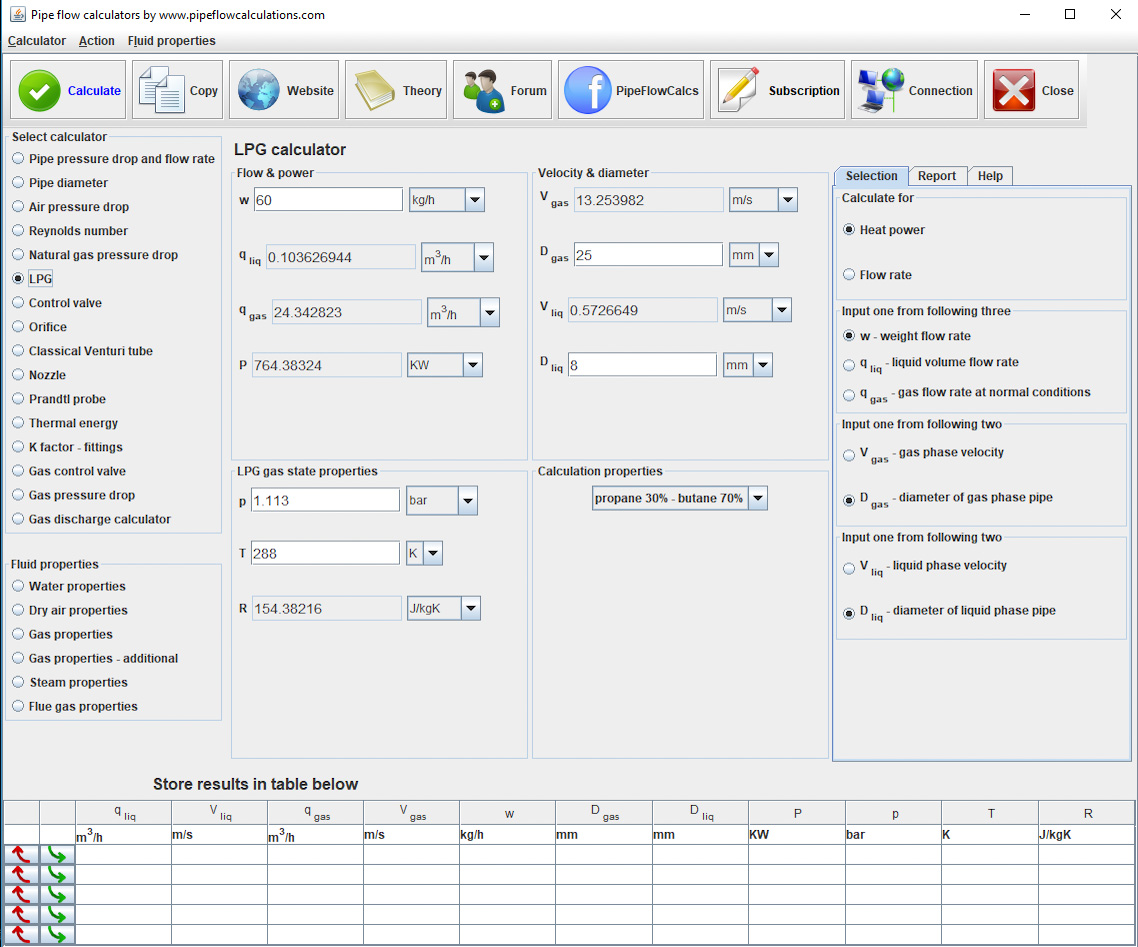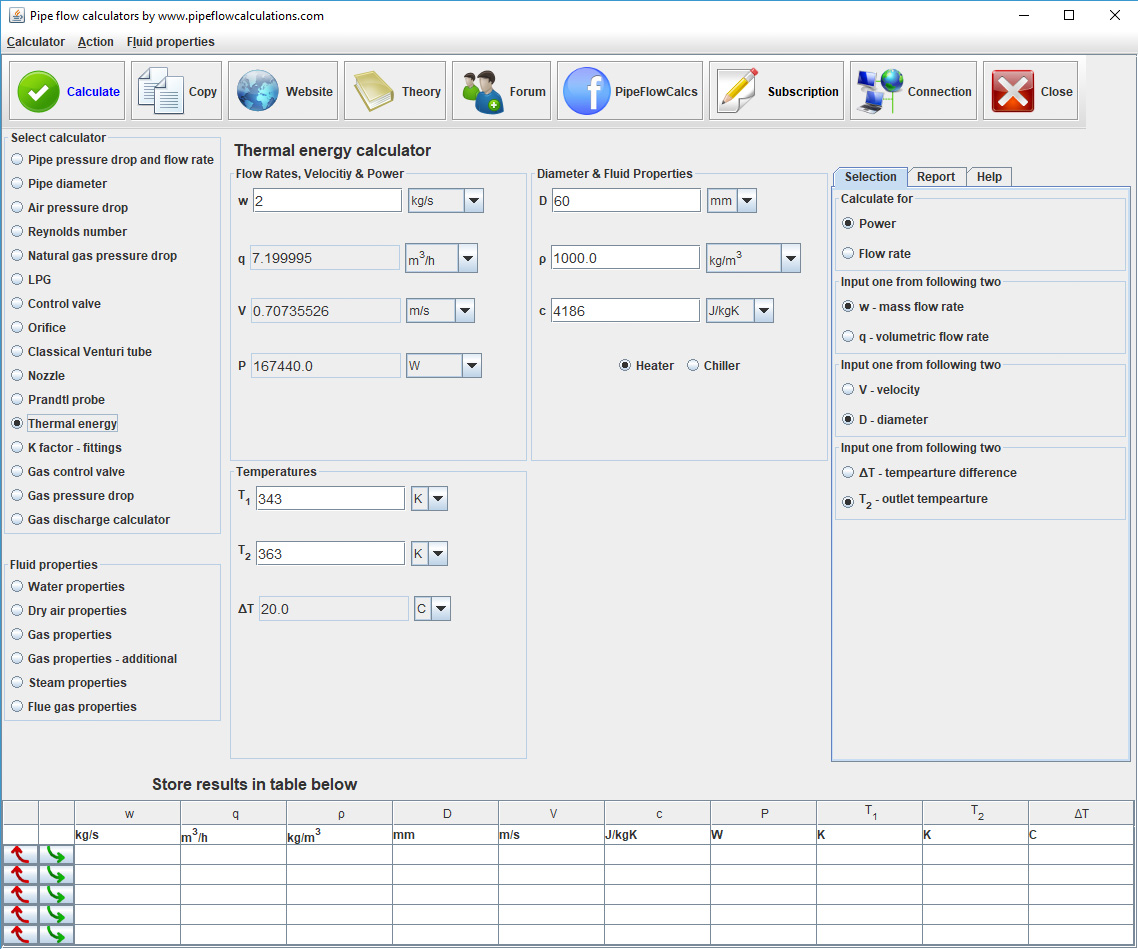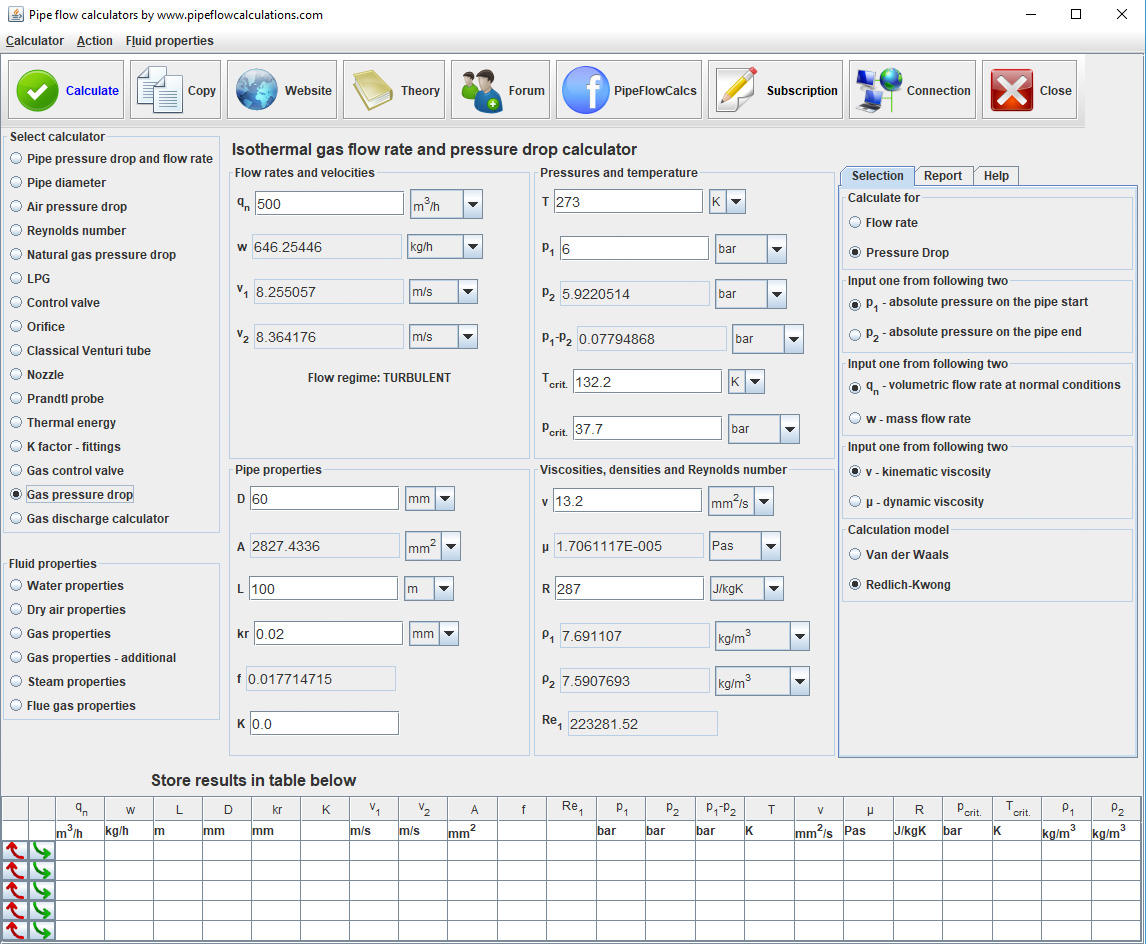# Combustion heat energy calculator

## fuel energy to heat power conversion

### online since 2014## Registration and subscription

Please login for full calculator service. Use the form on the top of the page.
Register an account if you don't have one.
Active subscription is required for full services.
Action
Calculation setup
Combustion heat power
Fuel heat values and density
Flow rates

# Calculator description

Read more about calculator application and check if calculator is suitable for your problem.

Also, read about calculation procedures, what you have to know for the calculations and what limitations apply.

# Registration and subscription

Subscribed users have access to the full service which includes:

• One click switch from metric to imperial units
• Export results in word or excel format
• Preview, copy and paste results from a web page to your text editor
• Send results to you email

## Calculator start selection

Read all about available deployments. In any way of utilizing calculator, Internet connection is required, for authentication at least.

## Issues and answers

Having problems with calculators usage? Read more about known issues and problems and way to solve it.

#### Description

q - Volume flow rate
Fuel flow rate in terms of units of volume per unit of time
ṁ - Mass flow rate
Fuel flow rate in terms of units of mass per unit of time
P - Power - heat power
Energy needed for combustion in unit of time
P1 - Actual power
Useful heat energy - power after reduction due to energy losses in energy conversion from combustion heat to, for example, hot water heat energy
LHV_V - Heat value
Heat energy created during combustion in units of volume
LHV_G - Heat value
Heat energy created during combustion in units of mass
ρ - Density of fuel
This value is only required if you want to calculate flow rate or heat power in different units (as per unit of mass or volume).
η - Efficiency
Energy losses coefficient ratio between actual heat power and combustion heat power that represents efficiency of energy conversion.

Efficiency coefficient is used to represent losses in energy conversion from combustion energy to energy available for end use like hot water heat energy from boiler. If you have specified hot water heat energy demand P1 and if you know boiler efficiency - η, calculator gives you required actual heat power from combustion like P1/ η. You can select not to use this feature by selecting η in Omit section.

#### Calculation setup

Select value to calculate. You should input values for not selected one.
P
heat power calculation
fuel flow rate calculation
LHV_V/LHV_G
fuel heat value calculation
Select value to input. Not selected one will be calculated.
P
heat power
P1
actual heat power
Select value to input. Not selected one will be calculated.
q
volume flow rate
mass flow rate
Select value to input. Not selected one will be calculated.
LHV_V
lower heat value in terms of volume
LHV_G
lower heat value in terms of mass
Use η in calculation
for calculation of actual energy after energy losses in combustion process
Use ρ in calculation
for conversion between mass and volume